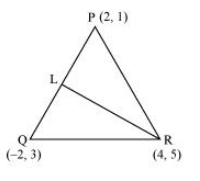# The vertices of ΔPQR are P (2, 1), Q (–2, 3) and R (4, 5).Question:

The vertices of $\triangle P Q R$ are $P(2,1), Q(-2,3)$ and $R(4,5)$. Find equation of the median through the vertex $R$.

Solution:

It is given that the vertices of $\triangle \mathrm{PQR}$ are $\mathrm{P}(2,1), \mathrm{Q}(-2,3)$, and $\mathrm{R}(4,5)$.

Let RL be the median through vertex R.

Accordingly, L is the mid-point of PQ.

By mid-point formula, the coordinates of point $L$ are given by $\left(\frac{2-2}{2}, \frac{1+3}{2}\right)=(0,2)$It is known that the equation of the line passing through points $\left(x_{1}, y_{1}\right)$ and $\left(x_{2}, y_{2}\right)$ is $y-y_{1}=\frac{y_{2}-y_{1}}{x_{2}-x_{1}}\left(x-x_{1}\right)$.

Therefore, the equation of $\mathrm{RL}$ can be determined by substituting $\left(x_{1}, y_{1}\right)=(4,5)$ and $\left(x_{2}, y_{2}\right)=(0,2)$.

Hence, $y-5=\frac{2-5}{0-4}(x-4)$

$\Rightarrow y-5=\frac{-3}{-4}(x-4)$

$\Rightarrow 4(y-5)=3(x-4)$

$\Rightarrow 4 y-20=3 x-12$

$\Rightarrow 3 x-4 y+8=0$

Thus, the required equation of the median through vertex $\mathrm{R}$ is $3 x-4 y+8=0$